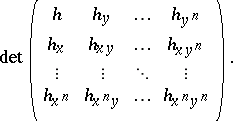# D'Alembert equation for finite sum decompositions

Consider the decomposition of a function $h ( x , y )$ into a finite sum of the form

\begin{equation*} h ( x , y ) = \sum _ { k = 1 } ^ { n } f _ { k } ( x ) g _ { k } ( y ). \end{equation*}

For sufficiently smooth $h$, a necessary condition for such a decomposition involves determinants of the formThese determinants were introduced in [a6] and [a7], and a correct formulation of the sufficient condition was given in [a3]; see also [a4].

A sufficient and necessary condition for not sufficiently smooth functions $h ( x , y )$ defined on arbitrary (even discrete) sets without any regularity conditions was formulated in [a3], [a4] by introducing a new, special matrix

\begin{equation*} \left( \begin{array} { c c c c } { h ( x _ { 1 } , y _ { 1 } ) } & { h ( x _ { 1 } , y _ { 2 } ) } & { \dots } & { h ( x _ { 1 } , y _ { n } ) } \\ { h ( x _ { 2 } , y _ { 1 } ) } & { h ( x _ { 2 } , y _ { 2 } ) } & { \dots } & { h ( x _ { 2 } , y _ { n } ) } \\ { \vdots } & { \vdots } & { \ddots } & { \vdots } \\ { h ( x _ { n } , y _ { 1 } ) } & { h ( x _ { n } , y _ { 2 } ) } & { \dots } & { h ( x _ { n } , y _ { n } ) } \end{array} \right); \end{equation*}

Several authors have dealt with problems concerning decompositions of functions of several variables and similar questions, see, e.g., [a1], [a2], [a8]. However, several open problems in this area remain (as of 2000), e.g.: find a characterization of functions $h ( x , y )$ of the form